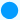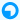Math Calculator

## Circle CalculatorDiameter of Circle: [ 2r ] Enter the radius = Diameter of Circle =Area of Circle: [πr² ] Enter the radius = Area of Circle =Circumference of Circle:[ 2πr ] Enter the radius = Circumference of Circle =Area of Sector: [ ½×arc×(angle in degree) ] Enter the radius = Enter the angle = Result Area =